Display Accessibility Tools

## Accessibility Tools

###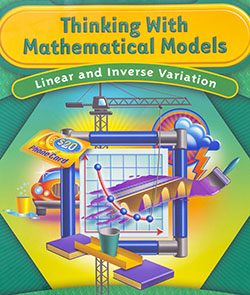Thinking with Mathematical Models

In Thinking With Mathematical Models students will study, compare and generalize two very different patterns relating two variables. In particular, they will learn how to:

• Recognize linear and non-linear patterns in tables and graphs and describe those patterns using words and symbolic expressions;
• Write equations to express linear patterns appearing in tables, graphs, and problem "stories";
• Solve linear equations;
• Model situations with inequalities;
• Write equations describing inverse variation;
• Use linear and inverse equations to solve problems and to make predictions and decisions.

###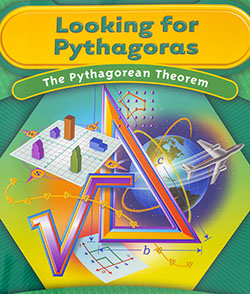Looking for Pythagoras

In Looking for Pythagoras students explore an important relationship about right triangles that connects algebra and geometry, called the Pythagorean Theorem. They learn to:

• Relate the area of a square to the length of a side of the square;
• Estimate square roots;
• Develop strategies for finding the distance between two points on a coordinate grid;
• Understand and apply the Pythagorean Theorem;
• Use the Pythagorean Theorem to solve a variety of problems.

###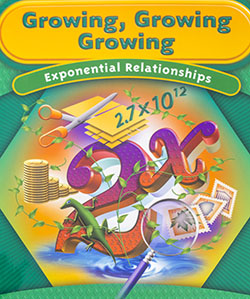Growing, Growing, Growing

Growing, Growing, Growing was created to help students make sense of exponential relationships. In this unit students will learn to:

• Recognize situations where one variable is an exponential function of another variable;
• Recognize the connections between exponential equations and growth patterns in tables and graphs of those relations;
• Construct equations to express exponential patterns that appear in data tables, graphs, and problem conditions;
• Understand and apply the rules for operating on numerical expressions with exponents;
• Solve problems about exponential growth and decay in a variety of situations such as sciences or business;
• Compare exponential and linear relationships.

###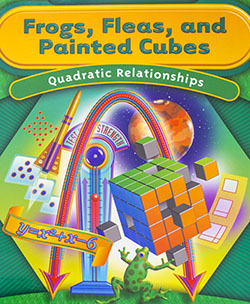Frogs, Fleas, and Painted Cubes

In Frogs, Fleas, and Painted Cubes students explore an important type of non-linear relationship, quadratic functions. The unit should help students to:

• Recognize the patterns of change for quadratic relationships in a table, graph, equation, and problem situation;
• Construct equations to express quadratic relationships that appear in tables, graphs and problem situations;
• Recognize the connections between quadratic equations and patterns in tables and graphs of those relationships;
• Use quadratic tables, graphs, and equations to locate maximum and minimum values of a dependent variable and the x- and y-intercepts and other important features of parabolas, the graphs of quadratic relationships;
• Recognize equivalent symbolic expressions for the dependent variable in quadratic relationships;
• Use the distributive property to write equivalent quadratic expressions in factored form or expanded form;
• Use tables, graphs, and equations of quadratic relations to solve problems in a variety of situations from geometry, science, and business;
• Compare properties of quadratic, linear, and exponential relationships.

###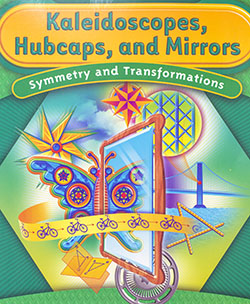Kaleidoscopes, Hubcaps, and Mirrors

In Hubcaps, Kaleidoscopes and Mirrors students investigate symmetries and transformations. This unit will help them to:

• Understand important properties of symmetry;
•
• Recognize and describe symmetries of figures;
• Use tools to examine symmetries and transformations;
• Create figures with specified symmetries;
• Identify basic design elements that can be used to replicate a given design;
• Perform symmetry transformations of figures, including reflections, translations, and rotations;
• Examine and describe the symmetries of a design created from a figure and its image(s) under a symmetry transformation;
• Give precise mathematical directions for performing reflections, rotations, and translations;
• Draw conclusions about a figure, such as measures of sides and angles, lengths of diagonals, or intersection points of diagonals, based on what symmetry or symmetries the figure has;
• Understand that figures with the same shape and size are congruent;
• Use symmetry transformations to explore whether two figures are congruent;
• Give examples of minimum sets of measures of angles and sides that will guarantee that two triangles are congruent;
• Use congruence of triangles to explore congruence of two quadrilaterals;
• Use symmetry and congruence to deduce properties of figures;
• Write coordinate rules for specifying the image of a general point (x, y) under particular transformations;
• Learn to appreciate the power of transformational geometry to describe motions, patterns, designs and properties of shapes in the real world

###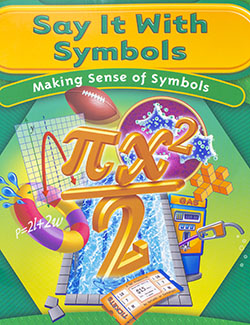Say It With Symbols

Say It With Symbols was created to help students make sense of symbolic representations. In this unit students learn to:

• Model situations with symbolic statements;
• Write equivalent expressions;
• Determine if different symbolic expressions are mathematically equivalent;
• Interpret the information equivalent expressions represent in a given context;
• Determine which equivalent expression to use to answer particular questions;
• Solve linear equations involving parentheses;
• Solve quadratic equations by factoring;
• Use equations to make predictions and decisions;
• Scan an equation to make estimates of the patterns that would emerge in tabular or graphic representations;
• Understand how and when symbols should be used to display relationships, generalizations, and proof.

###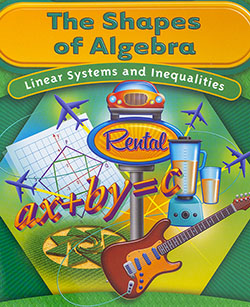Shapes of Algebra

The Shapes of Algebra was designed to help students capitalize on the strong connections between algebra and geometry in order to extend students understanding and skill in several significant aspects of those two key strands in the middle grades curriculum:

• Create and use equations of circles
• Determine if lines are parallel or perpendicular by looking at patterns in their graphs, vertex coordinates, and equations
• Find midpoints of line segments and use midpoint relationships to deduce further properties of triangles and quadrilaterals
• Write inequalities that satisfy given situations
• Find solutions to inequalities when given a graph or an equation
• Solve linear systems and find intersections of lines and equations through graphing, combination, and substitution
• Choose strategically among the three algebraic methods studied, which to use for a particular system of linear equations
• Write linear inequalities in two variables to match constraints in problem conditions
• Graph linear inequalities in two variables and systems of such conditions and use the results to solve problems

###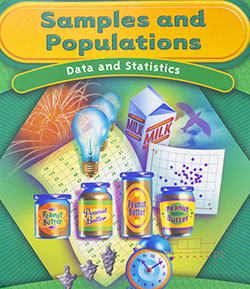Samples and Populations

In the unit Samples and Populations students collect, organize and analyze data. They learn to:

• Use the process of statistical investigation to explore problems;
• Choose appropriate samples from populations and use information from samples to draw conclusions about populations;
• Explore the influence of sample size and sample selection processes in trying to obtain a sample which is likely to be predictive about the population
• Apply concepts from probability to select random samples from populations
• Compare sample distributions using measures of center (mean, median), measures of spread (range, least to greatest data values, percentiles), and data displays that group data (histograms, box-and-whisker plots)
• Explore relationships between paired values of numerical attributes

### Clever Counting (CMP1)

The unit Clever Counting was developed to help students:

• Recognize which counting techniques apply;
• Construct organized lists of outcomes for complex processes and uncover patterns that help in counting the outcomes of those processes
• Use diagrams, tables, and symbolic expressions to organize examples in listing and counting tasks;
• Analyze the usefulness of counting trees and use counting trees;
• Use mental arithmetic to make estimates in multiplication and division calculations;
• Invent strategies for solving problems that involve counting;
• Analyze counting problems that involve choices in various contexts;
• Differentiate among situations in which order does and does not matter, an in which repeats are and are not allowed;
• Analyze the number of paths through a network;
• Compare the structure of networks with problems involving combinations;
• Create networks that satisfy given constraints;
• Apply thinking and reasoning skills to an open-ended situation in which assumptions must be made, and create a persuasive argument to support a conjecture;